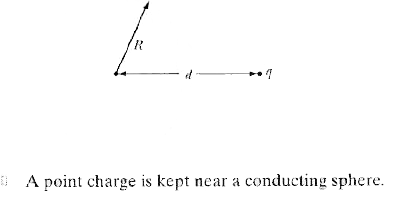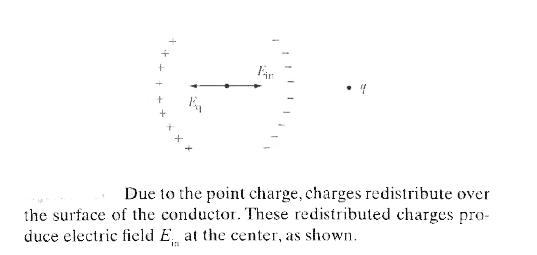# Find the electric field at the center of the sphere due to the induced charges on the surface of the sphere, as shown in Fig. 22-30.##### Updated On: 27-06-2022

Text Solution

Solution
KEY IDEA
Here, we note that the induced charges will form a certain pattern on the surface of the sphere which cannot be predicted. But we can find the electric field due to q at any point. The net electric field inside the conductor is zero. This is produced by the induced charged and the external charge.
Calculations: At the center this electric field due to q in the positive direction will be (Fig. 22-31):
EQ=q4πε0d2.Since the net electric field at the center has to be zero.
Einduced+EQ=0Einduced=q4πε0d2.
Here, the charges will be induced on the surface of the sphere, but they will not be uniformly distributed. So, we cannot apply the formula for the electric field due to a uniformly charged spherical shell.
Learn: When we say the electric field is zero, this implies that electric field should be zero inside the core of a solid metal. These are the points where the free electrons are present within the lattice. These free electrons should not experience any force.